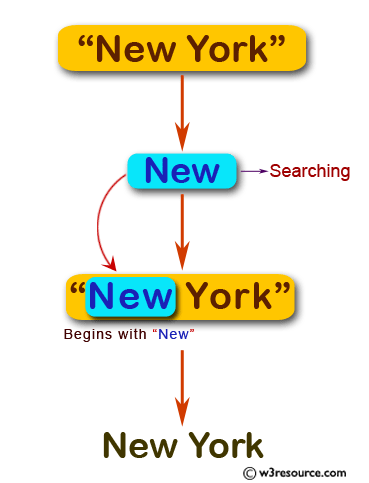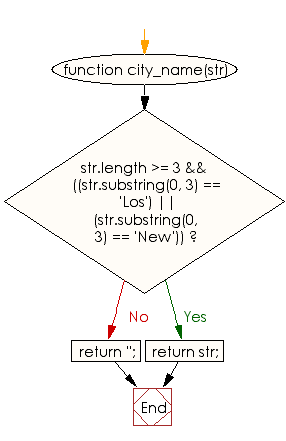# JavaScript: Display the city name if the string begins with "Los" or "New" otherwise return blank

## JavaScript Basic: Exercise-66 with Solution

Write a JavaScript program to display the city name if the string begins with "Los" or "New" otherwise return blank.

Pictorial Presentation:Sample Solution:

HTML Code:

``````<!DOCTYPE html>
<html>
<meta charset="utf-8">
<meta name="viewport" content="width=device-width">
<title>JavaScript program to display the city name  if the string begins with “Los” or “New” otherwise return blank.</title>
<body>

</body>
</html>
```
```

JavaScript Code:

``````function city_name(str) {
if (str.length >= 3 && ((str.substring(0, 3) == 'Los')
|| (str.substring(0, 3) == 'New')))

{
return str;
}

return '';
}

console.log(city_name("New York"));
console.log(city_name("Los Angeles"));
console.log(city_name("London"));
```
```

Sample Output:

```New York
Los Angeles
```

Flowchart:ES6 Version:

``````function city_name(str) {
if (str.length >= 3 && ((str.substring(0, 3) == 'Los')
|| (str.substring(0, 3) == 'New')))

{
return str;
}

return '';
}

console.log(city_name("New York"));
console.log(city_name("Los Angeles"));
console.log(city_name("London"));
``````

Live Demo:

What is the difficulty level of this exercise?

Test your Programming skills with w3resource's quiz.

﻿

## JavaScript: Tips of the Day

Returns the sum of the powers of all the numbers from start to end (both inclusive)

Example:

```const sumPower = (end, power = 2, start = 1) =>
Array(end + 1 - start)
.fill(0)
.map((x, i) => (i + start) ** power)
.reduce((a, b) => a + b, 0);
console.log(sumPower(10)); // 385
console.log(sumPower(10, 3)); // 3025
console.log(sumPower(10, 3, 5)); // 2925
```

Output:

```385
3025
2925
```